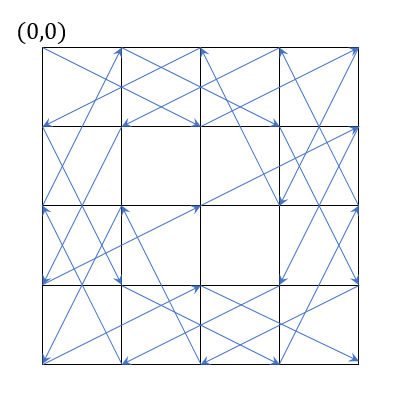Fenrier Lab

# 算法题：棋盘行走public class Node{
public final int x;
public final int y;
private List<Node> neighbors = new ArrayList<>();
private boolean hasVisited = false;
public Node(int x, int y){
this.x = x;
this.y = y;
}
}
public List<Node> getNeighbors(){
return neighbors;
}
public boolean hasVisited(){
return hasVisited;
}
public void visit(){
hasVisited = true;
}
public void reset(){
hasVisited = false;
}
public String toString(){
return "(" +x+","+y+ ")";
}
}


List<Node> nodes = new ArrayList<>();
for(int i = 0; i < m; i++) {
for(int j = 0; j < n; j++) {
}
}


$(x-2,y-1)\,,(x-2,y+1)\\(x-1,y-2)\,,(x-1,y+2)\\(x+1,y-2)\,,(x+1,y+2)\\(x+2,y-1)\,,(x+2,y+1)$

for (Node node : nodes) {
List<Node> nbs = calcNeighborsOf(node);
for (Node nb : nbs) {
}
}


public void dfs(Node start){
List<Node> visited = new ArrayList<>();
dfs(start, visited);
}
private void dfs(Node current, List<Node> visited){
current.visit();
boolean end = true;
for (Node node : current.getNeighbors()) {
if(!node.hasVisited()) {
end = false;
dfs(node, visited);
}
}
if(end) {
if(visited.size() == m * n) {
System.out.println(visited);
}
}
current.reset();
visited.remove(visited.size() - 1);
}


[(0,0), (1,2), (0,4), (2,3), (0,2), (1,0), (3,1), (4,3), (2,4), (0,3), (1,1), (3,0), (2,2), (1,4), (3,3), (4,1), (2,0), (0,1), (1,3), (3,4), (4,2), (2,1), (4,0), (3,2), (4,4)]